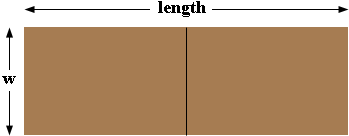SEARCH HOMEMath Central Quandaries & QueriesQuestion from alysia: A pig farmer wants to build two adjacent pens using 96 feet of fencing. He wants rectangular pens that share the short side and the overall length is to be six more than twice the width. What should be the dimensions of the overall pen?Alysia,

Draw a diagram.I let the width be w feet. The problem states "the overall length is to be six more than twice the width". What is twice the width? What is 6 more than twice the width? This is the length.

Look at the diagram again to determine the amount of fencing needed. You need three pieces w feet long and two pieces as long as the length you calculated above. Add the lengths of these 5 pieces together and this must be 96 feet. Solve for w.

I hope this helps,
PennyMath Central is supported by the University of Regina and The Pacific Institute for the Mathematical Sciences.